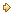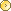Visitors Online: 77 | Sunday 26th May 2019

CBSE Guess > Papers > Question Papers > Class XII > 2006 > Economics > Outside Delhi Set - I

Economics — 2006 (Set I—Outside Delhi)

SECTION - A

Q. 1. Answer the following questions: (1 x 4 = 4)

1. When is a good called a ‘normal good’?
2. Define fixed cost.
3. Define marginal revenue.
4. Price elasticity of supply of a good is 0.8. Is the supply ‘elastic’ or ‘inelastic’, and why?

Q. 2. Define marginal utility. State the law of diminishing marginal utility. (3)

Q. 3. State any three factors that affect the price elasticity of demand of a commodity (3)

Q. 4. What is meant by returns to scale? State the reasons for increasing returns to scale. (3)

Q. 5. State any three causes of a leftward shift of supply curve. (3)

Q. 6. When the price of a commodity rises from Rs. 10 to Rs. 11 per unit, its quantity supplied rises by 100 units. Its price elasticity of supply is 2. Calculate its quantity supplied at the increased price. (4)

Q. 7. What will be the effect of the following changes in total revenue on marginal revenue? (4)

1. Total revenue increases at a decreasing rate.
2. Total revenue increases at a constant rate.

Q. 8. Draw a production possibility curve. What does a point below this curve indicate? Explain. (4)

Or

Explain the problem of ‘what to produce’ with the help of an example.

Q. 9. Draw the average revenue curve of a firm under

1. monopoly and
2. Perfect competition. Explain the difference in these curves, if any. (4)

Q. 10. Calculate total cost and average variable cost of a firm at each given level of output from its cost schedule given below. (6)

 Output (Units) Average fixed cost (Rs.) Marginal cost (Rs.) 1 60 32 2 30 30 3 20 28 4 15 30 5 12 35 6 10 43

Q. 11. Define market demand. State the factors that affect it. (6)

Q. 12. How will an increase in the income of the buyers of an ‘inferior good’, affect its equilibrium price and equilibrium quantity? Explain with the help of a diagram. (6)

Or

At a given price of a commodity, there is excess supply. Is it an equilibrium price? If not, how will the equilibrium price be reached? (Use diagram)

SECTION - B

Q. 13. Answer the following questions: (1 x 4 = 4)

1. In a government budget, primary deficit is Rs. 10,000 crores and interest payment is Rs. 8,000 crores. How much is the fiscal deficit?
2. Give two examples of macro-economic variables.
3. Define macro-economics.
4. When will balance of trade show a surplus?

Q. 14. From the following data calculate ‘gross value added at factor cost’: (3)

 (Rs. in lakhs) Net indirect tax Purchase of intermediate products Purchase of machines Sales Consumption of fixed capital Change in stock 20 120 300 250 20 30

Q. 15. What is meant by ‘investment multiplier’? Explain the relationship between marginal propensity to consume and investment multiplier. (3)

Q. 16. Define average propensity to save and marginal propensity to save. Can the value of average propensity to save be negative? Give reasons for your answer. (3)

Q. 17. State the basis of classifying government receipts into revenue receipts and capital receipts. Give an example of each. (3)

Q. 18. How does a central bank perform the function of controllerof credit? (4)

Or

Explain the meaning and any one function of a commercial bank.

Q. 19. Giving reasons categories the following into revenue expenditure and capital expenditure: (4)

1. Subsidies
2. Grants given to State Governments
3. Repayment of loans
4. Construction of school buildings

Q. 20. Define foreign exchange rate. Why does the demand for foreign exchange rise when its price falls? (4)

Q. 21. Explain any one problem faced in the barter system. How has money solved this problem? (4)

Q. 22. From the following data calculate net national product at factor cost by

1. income method, and
2. expenditure method: (3, 3)
 (Rs. in crores) Current transfers from rest of the world Government final consumption expenditure Wages and salaries Dividend Rent   Interest Net domesticcapital formation Profits Employers’ contribution to social security schemes Net exports Net factor income from abroad Consumption of fixed capital Private final consumption expenditure Net indirect tax 100 1,000 3,800 500 200 150 500 800 200 (-) 50 (-) 30 40 4,000 300

Or

Calculate (a) private income, and (b) personal disposable income from the following data:

 (Rs. in crores) Income from property and entrepreneurship accruing to government administrative departments Savings of non-departmental public enterprises Corporation tax Income from domestic product accruing to private sector Current transfers from government administrative departments Net factor income from abroad Direct personal taxes Indirect tax Current transfers from rest of the world Savings of private corporate sector 500100 80 4,500 200 (-) 50 150 220 80 500

Q. 23. Why must aggregate demand be equal to aggregate supply at the equilibrium level of income and output? Explain with the help of a diagram. (6)

Q. 24. Will the following factor incomes be a part of domestic factor income of India? Give reasons for your answer. (6)

1. Profit earned by foreign banks from their branches in India.
2. Salary received by Indian residents, working in American embassy in India.
3. Profits earned by an Indian company from its branch in Singapore.
4. Compensation of employees given to residents of China working in Indian embassy in China.

 Economics 2006 Question Papers Class XII Delhi Outside Delhi Compartment Delhi Compartment Outside DelhiSet ISet ISet ISet I

 CBSE 2006 Question Papers Class XIIEnglishSociologyFunctional EnglishPsychologyMathematicsPhilosopyPhysicsComputer ScienceChemistryEntrepreneurshipBiologyInformatics PracticesGeographyMultimedia & Web TechnologyEconomicsBiotechnologyBusiness StudiesPhysical EducationAccountancyFine ArtsPolitical ScienceHistoryAgriculture